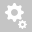##Recently viewed tickets# [PROPERTIES]  How to Use Section Stiffness Scale Factors ?

Section Stiffness Scale Factors
Properties > Section Manager > Stiffness

Stiffness scale factors can be applied to a particular section to manipulate(increase/decrease) the sectional stiffness data considered for the analysis. Useful in cases to perform Cracked Sectional Analysis, Rehabilitation, Stiffness deterioration etc.
For example, Let's consider a Simply Supported Beam of 20m length and rectangle (0.5mx0.5m) cross-section and apply 10kN/m UDL load and check the results with and without Section Stiffness Scale Factors. Here we will reduce the Iyy flexural stiffness by 50%.

Actual Section Stiffness are shown in below snapshot.Reduced Section Stiffness data can be applied by following the below procedure.As we do not have construction stages here and the Boundary group is "default", thereby these Stiffness scale factors will be applied for the entire analysis.

PFA two comparison models. Now Let's compare various results of both the models.

DeformationsThe scale factors defined here are applied to the calculations for displacements and member forces. For stress calculations, original section properties are used. However, the option for applying the Section Stiffness Scale Factors to Stress Calculations may be selected in Analysis > Main Control Data.Stress results:------------------------------------------------------------------------------------------------------------------------------------------------------------------------------------------

If Construction Stage Analysis is involved in the model, if we want to apply these factors in CS analysis, then we need to activate the Section Stiffness Scale Factors in the respective construction stages using relevant "boundary groups" option.------------------------------------------------------------------------------------------------------------------------------------------------------------------------------------------
Also for Tapered Sections when checking the results we need to be careful regarding its local axis direction. See below snapshot which explains the default direction. We can alter this from Main Control data.Thanks
Creation date: 11/17/2017 2:47 PM ()      Updated: 3/19/2018 5:41 AM ()